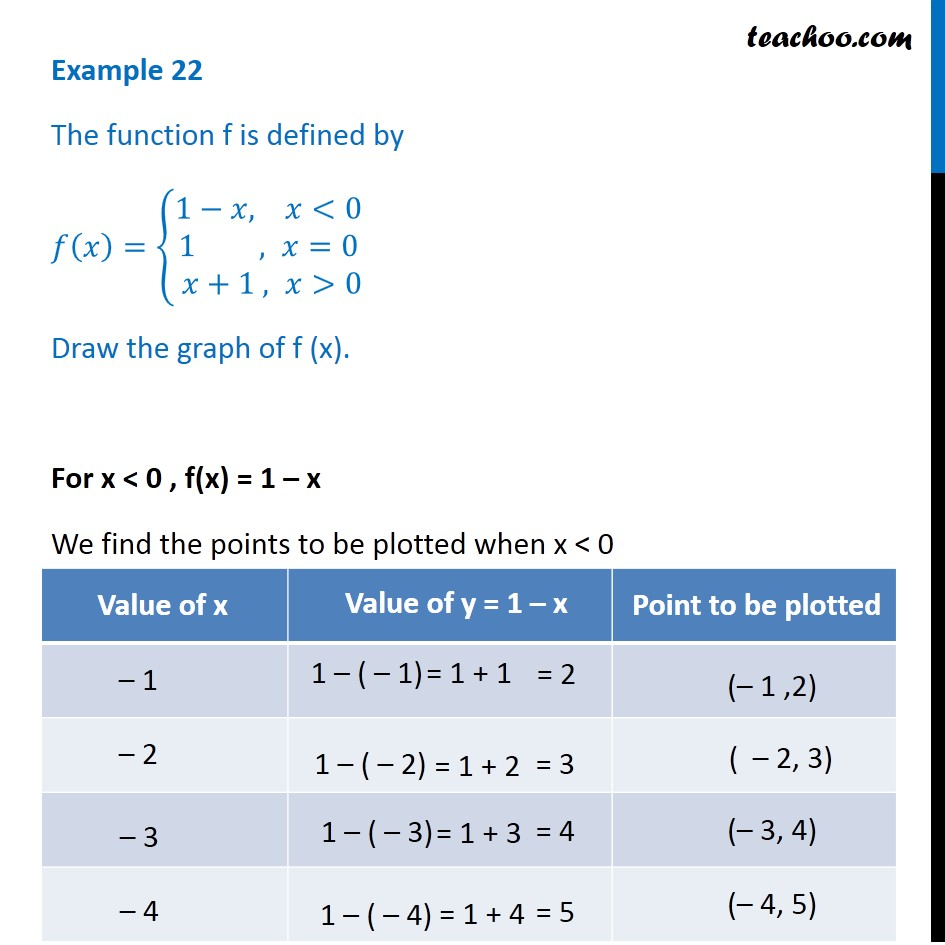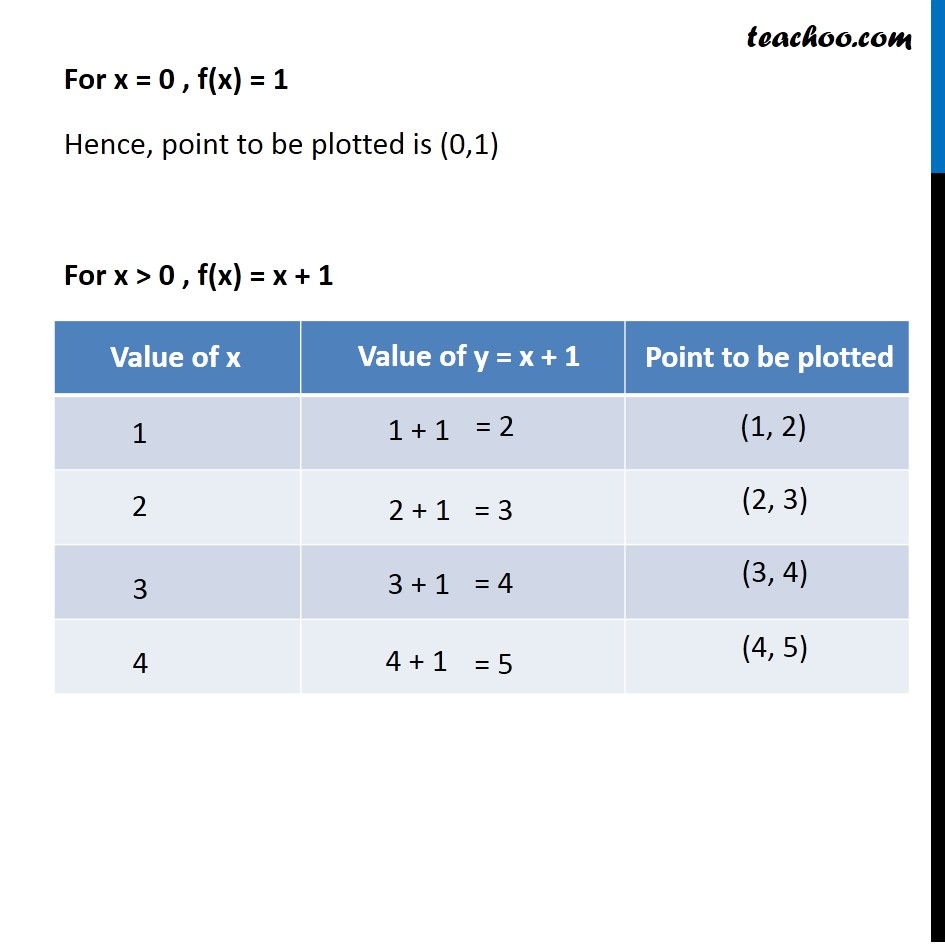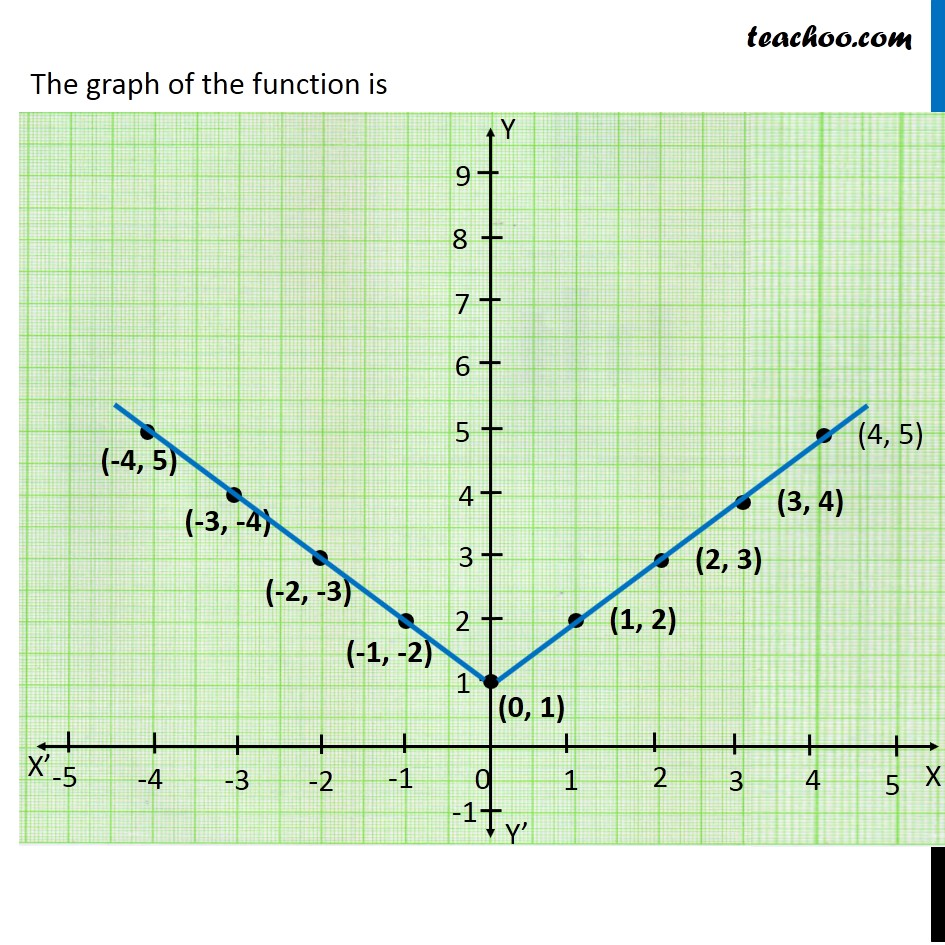Learn All Concepts of Chapter 2 Class 11 Relations and Function - FREE. Check - Relation and Function Class 11 - All Concepts1. Chapter 2 Class 11 Relations and Functions
2. Concept wise
3. Finding Domain and Range - By drawing graphs

Transcript

Example 22 The function f is defined by 𝑓(𝑥)={█(1−&𝑥, 𝑥<0@1 , 𝑥=0@ 𝑥+1 , 𝑥>0)┤ Draw the graph of f (x). For x < 0 , f(x) = 1 – x We find the points to be plotted when x < 0 For x = 0 , f(x) = 1 Hence, point to be plotted is (0,1) For x > 0 , f(x) = x + 1 We find the points to be plotted when x > 0 Hence we make a graph The graph of the function is

Finding Domain and Range - By drawing graphs